# Chapter 2 Theory

A theory –including a necessity theory– consists of four parts: concepts, propositions, focal unit and theoretical domain. The concepts are the variable parts of a theory that can increase or decrease. In NCA the independent concepts are called conditions and the dependent concepts outcomes. A proposition describes the causal relationship between the concepts. In NCA the causal relationship is a necessity relationship: ‘$$X$$ is necessary for $$Y$$.’ The focal unit is the object to which the theory applies: country, person, firm, project. etc. The theoretical domain is the set of cases of the focal unit where the theory is supposed to hold. A case is one particular example of the focal unit: a specific country, a specific person, etc.

The four characteristics of theory on which the nca.example data set of the NCA software is based are:

• Focal unit: country.

• Concepts:

• Independent concept (condition): Individualism.

• Dependent concept (outcome): Innovation performance.

• Proposition: Individualism is necessary for Innovation performance.

• Theoretical domain: all countries of the world.

In theory testing research, empirical data are used to test the propositions. The theoretical concepts are operationalized into variables that can be measured, the propositions are operationalized into hypotheses consisting of variables with their expected relationship, the focal unit becomes the unit of analysis, and cases are selected from the theoretical domain. For the case selection usually a population of cases is identified in the theoretical domain and a sample is taken from this population. From the sample a dataset is obtained with variables scores of the cases.

## 2.1 Formulation of necessity hypotheses

The propositions (or the operationalized hypotheses) are the core elements of a theory. They describe the expected causal relationships between the concepts. NCA starts with formulating a hypothesis that a condition is a necessary cause for the outcome. In some fields the existence of a causal necessity relationship is widely known and accepted. An example is the psychological theory that intelligence is necessary for creativity. This hypothesis was proposed in the 1960’s and is still widely around. Until recently, this theory could not be tested with a specific necessity method. When NCA became available it was possible to ensure theory-method fit by testing the hypothesis with NCA, as was done by .

However, most research areas do not have widely accepted necessity theories. Although necessity causality exists in virtually all research areas, and necessity statements are commonly made, necessity statements are usually not part of the theories. NCA gives the researcher and opportunity to build and test necessity theories.

Three complementary approaches can be used for for formulating necessary condition hypotheses. First, existing necessity statements in the literature can be identified. Although several necessity statements may use the word ‘necessity’ rather loosely as synonym for ‘important,’ many necessity statements refer to necessity logic and causality.

Second, when ‘important’ contributing causal factors for a outcome of interest have been identified with conventional methods, several of them could also be necessary conditions. For example, when a researcher formulates an additive theory (to be analysed with regression analysis) arguments are provided why a concept is an important contributor to an outcome. As a standard, the arguments imply sufficiency logic (‘$$X$$ produces $$Y$$’), but as an alternative, the relationship could also be described with necessity logic: an important factor that cannot be compensated for. Not only independent concepts but also mediator and moderator concepts in additive theories could be candidate necessary conditions. In other words, when current additive theories state that concepts are ‘important’ contributing concepts they may or may not (also) be necessary. But also contributing concepts that are considered ‘unimportant’ according to additive theories could be necessary.

Third, ‘critical’ and ‘bottleneck’ factors in practice could be candidate necessary conditions to be included in the theory. Practitioners commonly act upon ‘critical success factors’ or ‘must have’ factors (versus ‘nice to have’ factors). When such factor does not have the required level it can become a bottleneck on which the practitioner must act to ensure a desired outcome. Practitioners often have a ‘theory in use’ in which they argue why a factor is related to an outcome. Often the arguments imply necessity logic.

When a potential necessary condition is selected the researcher can do a ‘thought-experiment’ to test if necessity is possible. She can ask the question “Can I imagine cases with outcome $$Y$$, but without condition $$X$$?” When such cases are relatively common, the conclusion is that the condition is not necessary. When cases with the outcome $$Y$$ and without the condition $$X$$ are impossible or rare, the hypothesis may generally hold. The rare cases can be used to define the context where the hypothesis holds and where not, and thus to define the theoretical domain of the theory. Defining the theoretical domain –where the theory is supposed to hold– is important for all theories, because theories do rarely hold universally.

To illustrate the thought-experiment and the process of defining the theoretical domain, assume that a researcher wants to test the hypothesis ‘understanding English is necessary for reading this book.’ This statement is generally correct, but it is possible to imagine cases (persons) with the outcome (reading the book) but without the condition (not mastering English). Such exception is possible when someone translates the book in the language other than English, such that a reader who does not master English can read the translated book. This exception helps to specify the theoretical domain of the hypothesis: ‘persons reading the original version of book.’ Further exploration of exceptions can help to specify the theoretical domain. This process continues until the theoretical domain is precisely specified where the hypotheses is expected to hold virtually always. For testing this hypothesis, cases are selected from this specified theoretical domain. This can help to avoid sampling error (see section 3.7).

It is also possible that the necessary condition is rejected in the thought experiment because the condition is formulated too narrow. For example, ‘green apple’ is not necessary for an apple pie, and also red apple is not necessary for an apple pie. However, the higher-order construct (apple) is necessary. Then it would make sense to formulate the necessary condition hypothesis with the higher order concept. In general, attractive theories are supposed to be valid in a broad set of contexts, not only in very specific situations.

A hypothesis is usually only generally and semantically described as a theoretical statement. In NCA the common hypothesis is a qualitative theoretical statement like “X is necessary for Y” (in kind). A hypothesis usually is not described as a quantitative statement like “a specific level of X is necessary for a specific level of Y” (in degree). A hypothesis usually includes or implies a ‘direction’ of the causal relationship. In NCA four ‘directions’ are possible: the presence/high value of $$X$$ is necessary for the presence/high value of $$Y$$ (+ +), the absence/low value of $$X$$ is necessary for the presence/high value of $$Y$$ (- +), the presence/high value of $$X$$ is necessary for the absence/low value of $$Y$$ (+ -), and the absence/low value of $$X$$ is necessary for the absence/low value of $$Y$$ (- -). This is shown in Figure 2.1, where the empty space is in different corners.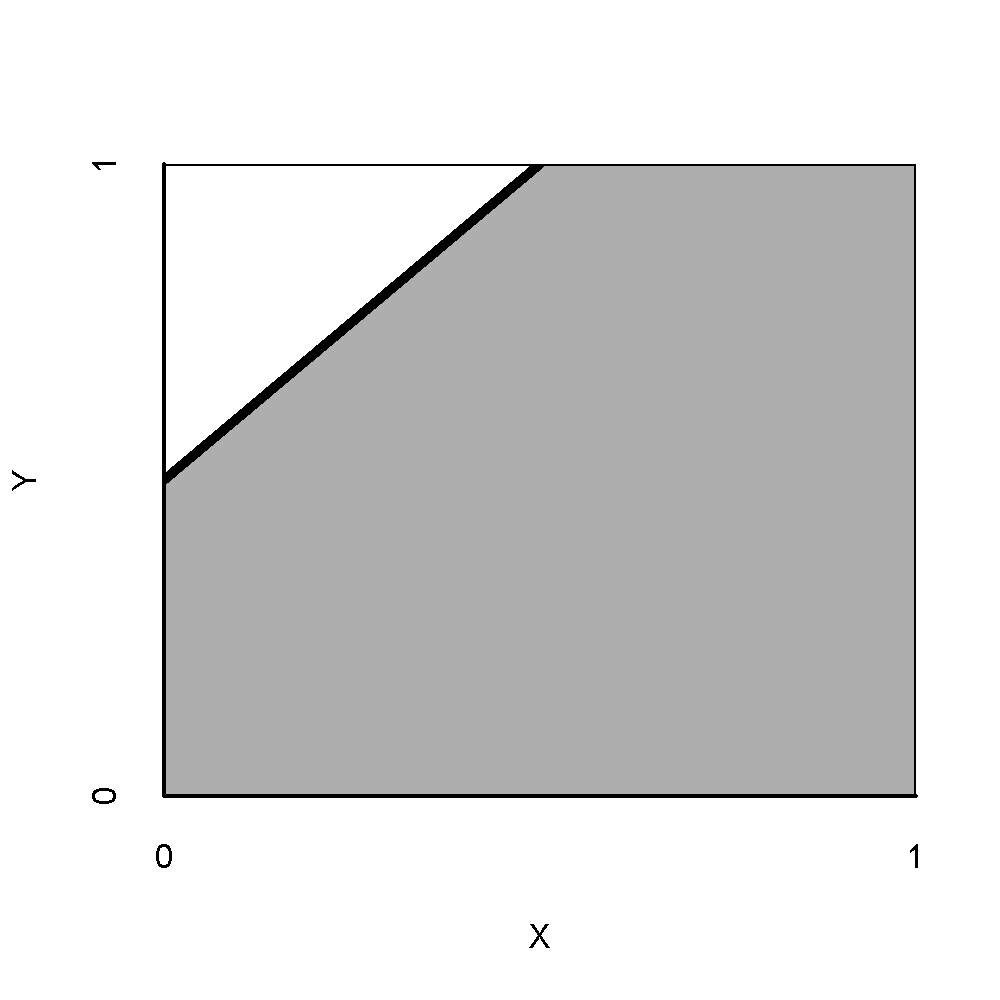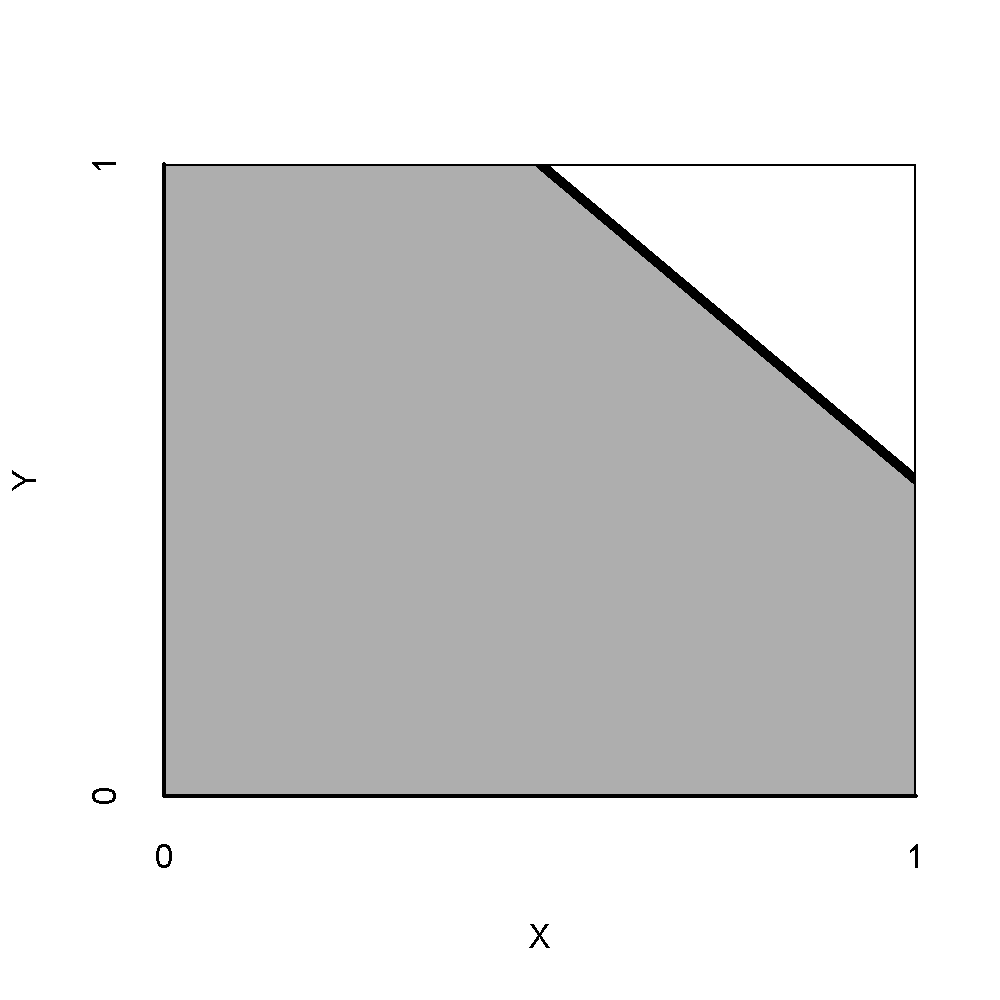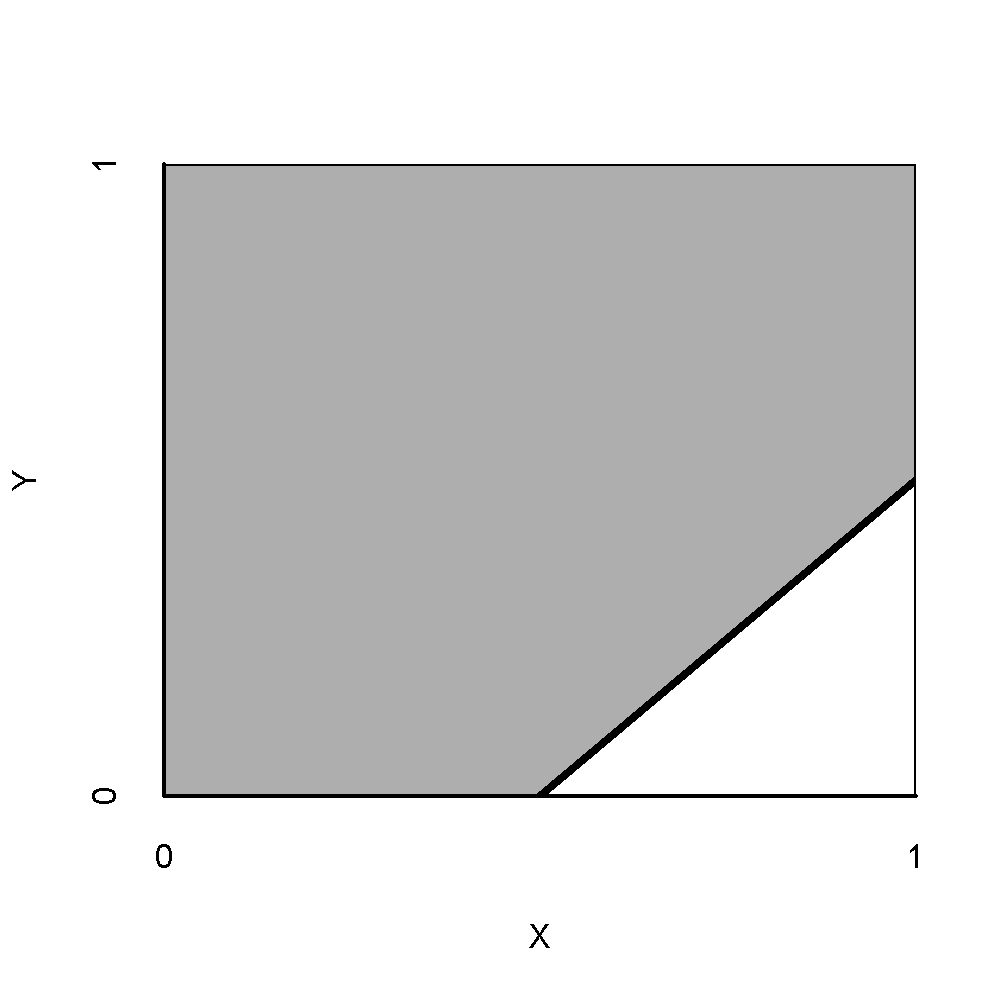Figure 2.1: ‘Direction’ of necessary conditions. Upper-left: a high value of $$X$$ is necessary for a high value of $$Y$$ (+ +). Upper-right: a low value of $$X$$ is necessary for a high value of $$Y$$ (- +). Lower-left: a high value of $$X$$ is necessary for a low value of $$Y$$ (+ -). Lower-right: a low value of $$X$$ is necessary for a low value of $$Y$$ (- -).

If the direction is not specified, the + + direction is implied. Note that in NCA the hypothesis is not described by a verb like in ‘$$X$$ increases $$Y$$’ or ‘$$X$$ has a positive effect on $$Y$$,’ which are common formulations to express sufficiency relationships. In NCA a noun or adverb is used to describe levels of $$X$$ and $$Y$$ as for example in ‘the absence of $$X$$ is necessary for the presence of $$Y$$’ or ‘low $$X$$ is necessary for high $$Y$$.’

## 2.2 Justification of necessity hypotheses

A theory is more than a set of hypotheses. It is also important to describe the mechanism why $$X$$ is necessary for $$Y$$. A first requirement for claiming causality is that condition occurs before the outcome: first the condition, then the outcome. Furthermore, the necessity relationship can be argued by answering three why questions:

• Why if $$Y$$ is present also $$X$$ is present?
• Why if $$X$$ is absent also $$Y$$ is absent?
• Why the absence of $$X$$ cannot be compensated by another concept?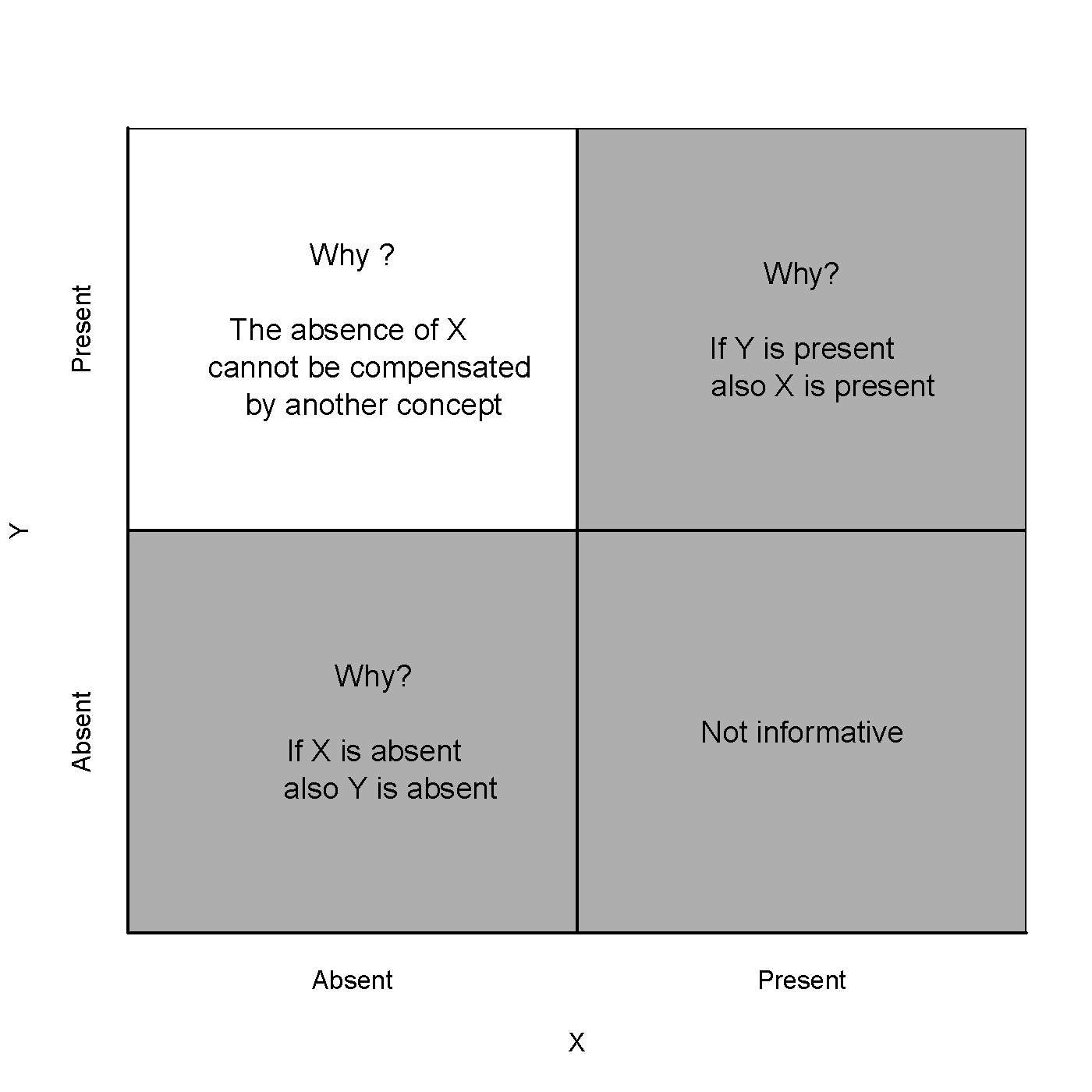Figure 2.2: Questions to justify a necessity hypothesis.

Figure 2.2 shows that these questions relate to three cells of the 2x2 contingency table that are compatible with the necessary condition hypothesis: where cases are possible or impossible when the necessary condition ‘the presence of $$X$$ is necessary for the presence of $$Y$$’ holds. Note that the lower right cell is not informative about necessity.

Describing the causal mechanism is often difficult. It is a creative process inspired by the three questions. The answers can be based on exploring and combining knowledge from the literature, from practice and from the researcher’s own experience. In the literature, researchers may partly use necessity arguments when describing the causal mechanism why an ‘important’ factor produces an outcome. Practitioners may tell stories why a ‘must have’ factor is ‘critical’ or a ‘bottleneck’ for the outcome and why he acts upon them for getting the outcome.

## 2.3 Types of necessity theories

distinguish two types of necessity theories. First, Pure necessity theories are theories that consist only of necessary condition relationships between $$X$$’s and $$Y$$’s. Because necessary conditions operate in isolation from the rest of the causal structure, such theories can be parsimonious with only one or a few necessary conditions. An example of a pure necessity theory with one condition and one outcome is the early theory of that intelligence is ‘necessary-but-not-sufficient’ for creativity. Shortly after NCA was introduced , this theory was empirically tested with NCA by . Other examples of pure necessity theories that have been proposed and tested with NCA and that are described in well-cited articles are theories about necessary conditions for lean manufacturing , for workplace spirituality , and innovation in buyer-supply relationships . Also the established Theory of Planned Behavior (TPB) has been revisited by from the perspective of necessity, showing with NCA that intention is a necessary but not sufficient condition for behavior. Other established theories could be revisited as well, and tested with NCA. For example, the Resource Based View of the firm (RBV) is a framework that is often described in terms of necessity (e.g., Wernerfelt, 1984) but has not yet been tested accordingly. One theory about the necessary conditions for success of start-up firms was developed based on literature and common beliefs, but this necessity theory was rejected after empirical testing with NCA .

Second, Embedded necessity theories are theories that consist of a mix of necessity relations and common additive relations where all factors are supposed to contribute to producing the outcome, but some factors (additionally) may also be necessary. These theories are usually complex as their main goal is to describe how the outcome is produced (sufficiency). One of the first embedded necessity theories is an extension of the Technology Acceptance Model (TAM). first described TAM as a structural (sufficiency) model including the classical antecedents ‘perceived usefulness’ and ‘perceived ease of use’ and analysed this model with Partial Least Squares Structural Equation Modeling (PLS-SEM). Subsequently, the relations were described as necessity conditions, and analysed with NCA. The results show that, for example the two classical antecedents not only help to produce technology acceptance on average, but they are also necessary.

## 2.4 Two empty corners

It is possible that a researcher formulates a theory that a single factor has two necessity relations with the outcome at the same time, implying that more than one corner is empty.

First, a researcher may theorize that an optimum (not low, not high) level of $$X$$ is necessary for a high level of $$Y$$. Then the upper left and the upper right corners are expected to be empty (Figure 2.3, left). Similarly, a researcher may theorize that an optimum (not low, not high) level of $$X$$ is necessary for a low level of $$Y$$. Then the lower left and the lower right corners are expected to be empty (Figure 2.3, right).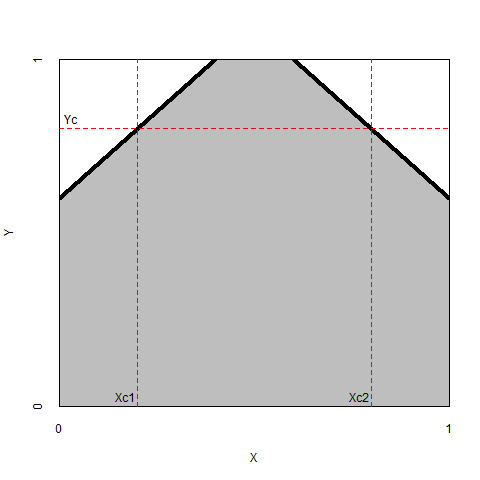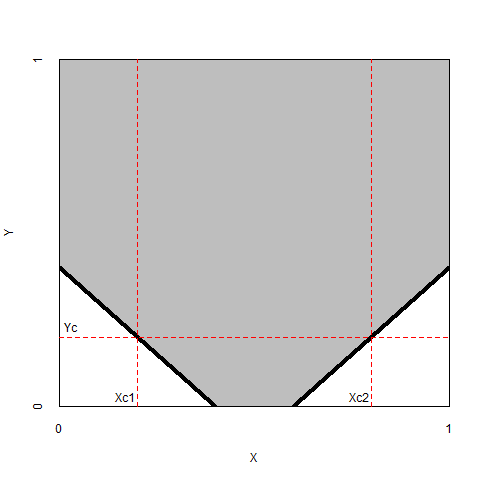Figure 2.3: Left: The necessity of an optimum level of $$X_{c1} < X < X_{c2}$$ for a high level of $$Y = Y_c$$. Right: The necessity of an optimum level of $$X_{c1} < X < X_{c2}$$ for a low level of $$Y = Y_c$$.

It is also possible that a researcher theorizes that a high level of $$X$$ is necessary for an extreme (low or high) level of $$Y$$. Then the upper left and the lower left corners are expected to be empty (Figure 2.4, left). Similarly, a researcher may theorize that a low level of $$X$$ is necessary for an extreme (low or high) level of $$Y$$. Then the upper right and the lower right corners are expected to be empty (Figure 2.4, right).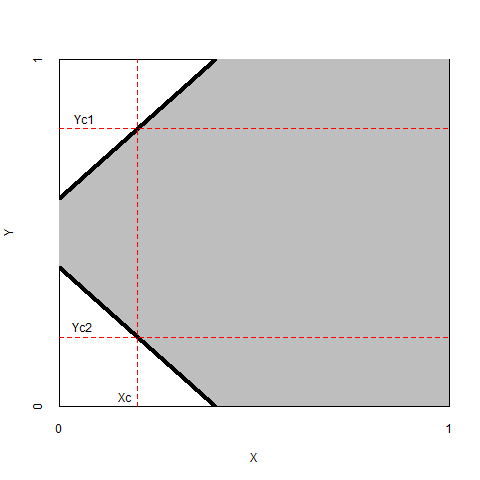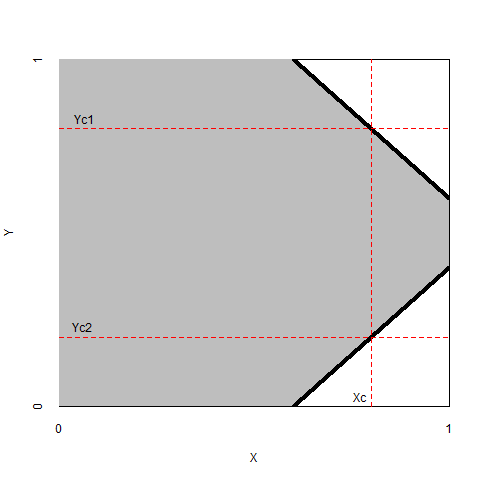Figure 2.4: Left: The necessity of a high level of $$X > X_c$$ for an extreme (low or high) level of $$Y = Y_{c1}$$ or $$Y = Y_{c2}$$. Right: The necessity of a low level of $$X < X_c$$ for an extreme (low or high) level of $$Y = Y_{c1}$$ or $$Y = Y_{c2}$$.

The final possible situation with two empty corners is that a researcher theorizes that a high level of $$X$$ is necessary for a high level of $$Y$$ and a low level of $$X$$ is necessary for a low level of $$Y$$ (Figure 2.5, left). Similarly, a researcher may theorize that a low level of $$X$$ is necessary for a high level of $$Y$$ and that a high level of $$X$$ is necessary for a low level of $$Y$$. Then two opposite corners are empty (Figure 2.5, right).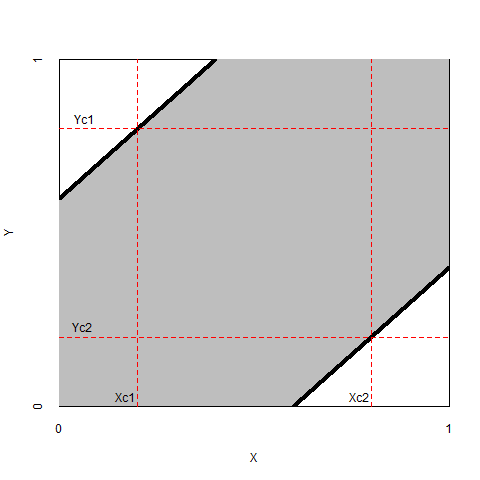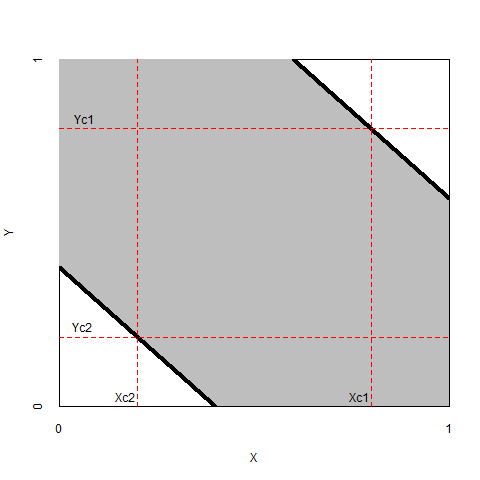Figure 2.5: Left: The necessity of a high level of $$X > X_{c1}$$ for a high level of $$Y = Y_{c1}$$, and the necessity of low level of $$X < X_{c2}$$ for a low level of \$Y = $$Y_{c2}$$. Right: The necessity of low level of $$X < X_{c1}$$ for a high level of $$Y = Y_{c1}$$, and the necessity of high level of $$X > X_{c2}$$ for a low level of $$Y = Y_{c2}$$.

In the NCA software, it is possible to do a combined statistical test of the two necessity relations of a single factor by using the argument effect_aggregation in the nca_analysis function. The software estimates the aggregate effect size, which is the sum of the effect sizes of the separate empty spaces, and its p value. This can be illustrated with the example that a country’s high level of Risk taking is necessary for a high level and sufficient for a low level of a country’s Innovation performance.

library(NCA)
data(nca.example)
#High level of Risk taking is necessary for high level of Innovation performance
model1 <- nca_analysis(nca.example,2,3, corner = 1, ceilings = "ce_fdh", test.rep = 10000)
## Preparing the analysis, this might take a few seconds...
## Do test for   : ce_fdh - Risk taking
Done test for : ce_fdh - Risk taking
model1
##
## --------------------------------------------------------------------------------
##             ce_fdh p
## Risk taking 0.309  0.101
## --------------------------------------------------------------------------------
nca_output(model1, plots = T, summaries = F)

#High level of Risk taking is sufficient for low level of Innovation performance
model2 <- nca_analysis(nca.example,2,3, corner = 4, ceilings = "ce_fdh", test.rep = 10000)
## Preparing the analysis, this might take a few seconds...
## Do test for   : ce_fdh - Risk taking
Done test for : ce_fdh - Risk taking
model2
##
## --------------------------------------------------------------------------------
##             ce_fdh p
## Risk taking 0.123  0.022
## --------------------------------------------------------------------------------
nca_output(model2, plots = T, summaries = F)

#High level of Risk taking is necessary for high level of Innovation performance and sufficient for low level of Innovation performance
model3 <- nca_analysis(nca.example,2,3, effect_aggregation = c(4), ceilings = "ce_fdh", test.rep = 10000)
## Preparing the analysis, this might take a few seconds...
## Do test for   : ce_fdh - Risk taking
Done test for : ce_fdh - Risk taking
model3
##
## --------------------------------------------------------------------------------
##             ce_fdh p
## Risk taking 0.432  0.028
## --------------------------------------------------------------------------------

Model 1 tests the emptiness of the upper left corner; model 2 of the lower right corner and model 3 is the aggregate test. The results of the latter test shows that the combined necessity/sufficiency relationship of Risk taking for Innovation performance is not rejected.

## 2.5 The meaning of ‘necessary but not sufficient’

Figure 2.1 shows a single factor with a one necessity relationship, and Figures 2.3, 2.4, and 2.5 show a single factor with two necessity relationships. In all figures, consistent with necessity, there are no observations in the empty space and observations in the grey area. If the necessity relationship holds, and no observations were in the grey area, all observations would be exactly on the border line. Then, the necessary condition is also a sufficient conditions. However this is not realistic as single necessary conditions are seldom also sufficient. This implies that the words ‘but not sufficient’ can normally be added to all necessity statements.

For example, upper left Figure 2.1, shows a single factor with one necessary condition: only the upper left corner is empty. This corresponds to the statement “a high level of $$X$$ is necessary for a high level of $$Y$$.” If all points were on the border line (ceiling line) the condition is also sufficient resulting in the statement: “a high level of $$X$$ is necessary and sufficient for a high level of $$Y$$.” In practice, observations will appear below the ceiling line. This justifies the statement that “a high level of $$X$$ is necessary but not sufficient for a high level of $$Y$$.”

Figure 2.3, left is an example of a factor with two necessity relations, “a high level of $$X$$ is necessary for a high level of $$Y$$” and “a low level of $$X$$ is necessary for a high level of $$Y$$” (thus an optimum level of $$X$$ is necessary for a high level of $$Y$$). If all observations were on the two ceiling lines, the words ‘and sufficient’ could be added to the word ‘necessary.’ Again, in practice observations show up below the border lines such that the words “but not sufficient” can be added to the word ‘necessary.’ The same holds for any other necessity relationship shown in Figures 2.1, 2.3, 2.4, and 2.5.

Any necessity statement can be rephrased as a sufficiency statement by formulating its logical complement. For example, the upper left corner of Figure 2.3 left (ignoring the upper right corner) indicates that a high level of $$X$$ is necessary but not sufficient for a high value of $$Y$$. This can be reformulated as ‘the absence of a high level of $$X$$ is sufficient but not necessary for the absence of a high value of $$Y$$.’ This means that a level of $$X < X_{c1}$$ guarantees (is sufficient for) $$Y < Y_c$$.

Similarly, the lower right corner of Figure 2.3 right (ignoring the lower left corner) indicates that a low level of $$X$$ is necessary but not sufficient for a low value of $$Y$$. This can be reformulated as: ‘the absence of a low level of $$X$$ is sufficient but not necessary for the absence of a high level of $$Y$$.’ A value of $$X > X_{c2}$$ guarantees that $$Y > Y_c$$.

When a single factor has two necessary relationships, it is possible to use the necessity formulation for one necessary condition, and the sufficiency formulation for the other. For example, in Figure 2.5, left the double necessity formulation is: ‘a high level of $$X > X_{1c}$$ is necessary but not sufficient for a high level of $$Y = Y_{c1}$$,’ and ‘the absence or low level of $$X < X_{c2}$$ is necessary but not sufficient for the absence or low level of $$Y = Y_{2c}$$.’ When the necessity formulation is used for the upper left necessity relation, and the sufficiency formulation for the lower right necessity relation, the new equivalent statement is: ‘a high level of $$X > X_{1c}$$ is necessary but not sufficient for a high level of $$Y = Y_{c1}$$,’ and ‘a high level of $$X < X_{c2}$$ is sufficient but not necessary for a high level of $$Y = Y_{2c}$$.’

Note that dual statements cannot be combined into a single statement, because the two border lines have a different location. This means that for a given $$Y$$ there are different levels of $$X$$ (see Figures 2.3, and 2.5) and for a given $$X$$ there different levels of $$Y$$ (see Figures 2.4, and 2.5).

Sometimes, the situation in Figure 2.5, left is formulated ‘in kind’ as ‘$$X$$ is necessary and sufficient for $$Y$$,’ which implies that the upper left necessity relationship is formulated in terms of necessity, and the lower right in terms of sufficiency. However, this formulation is problematic when the actual necessity relationships are ‘in degree.’ The ‘in degree’ formulation of the situation is not: ‘a high level of $$X$$ is necessary and sufficient for a high level of $$Y$$,’ but instead: ‘$$X > X_{c1}$$ is necessary for $$Y = Y_{c1}$$ and $$X > X_{c2}$$ is sufficient for $$Y = Y_{c2}$$.’ There is no common $$X$$ that is necessary and sufficient for a common $$Y$$ and no common $$Y$$ for which a common $$X$$ is necessary and sufficient.

Only when the two borderlines overlap, the ‘in kind’ statement also holds in an ‘in degree’ situation: ‘a certain level of $$X$$ is necessary and sufficient for a certain level of $$Y$$.’ At best, the ‘in kind’ formulation could be re-formulated as ‘a high level of $$X$$ is necessary for a high level of $$Y$$ and sufficient for a not so high level of $$Y$$,’ or similar. The theoretical meaning is that a high level of $$X$$ is necessary for (enables) a high level of $$Y$$ (border line = ceiling line) and is sufficient for (ensures) a low level of $$Y$$ (border line = floor line).

### References

Arenius, P., Engel, Y., & Klyver, K. (2017). No particular action needed? A necessary condition analysis of gestation activities and firm emergence. Journal of Business Venturing Insights, 8, 87–92. https://doi.org/10.1016/j.jbvi.2017.07.004
Bokrantz, J., & Dul, J. (2022). Building and testing necessity theories in supply chain management. Journal of Supply Chain Management, in press. https://onlinelibrary.wiley.com/doi/10.1111/jscm.12287
Dul, J. (2016b). Necessary Condition Analysis (NCA) logic and methodology of “necessary but not sufficient” causality. Organizational Research Methods, 19(1), 10–52. https://doi.org/10.1177/1094428115584005
Dul, J., & Hak, T. (2008). Case study methodology in business research. Routledge. https://www.routledge.com/Case-Study-Methodology-in-Business-Research/Dul-Hak/p/book/9780750681964
Frommeyer, B., Wagner, E., Hossiep, C. R., & Schewe, G. (2022). The utility of intention as a proxy for sustainable buying behavior–a necessary condition analysis. Journal of Business Research, 143, 201–213. https://doi.org/10.1016/j.jbusres.2022.01.041
Garg, N. (2017). Workplace spirituality and employee well-being: An empirical exploration. Journal of Human Values, 23(2), 129–147. https://doi.org/10.1177/0971685816689741
Guilford, J. P. (1967). The nature of human intelligence. McGraw-Hill. https://www.worldcat.org/title/nature-of-human-intelligence/oclc/204270
Karwowski, M., Dul, J., Gralewski, J., Jauk, E., Jankowska, D. M., Gajda, A., Chruszczewski, M. H., & Benedek, M. (2016). Is creativity without intelligence possible? A necessary condition analysis. Intelligence, 57, 105–117. https://doi.org/10.1016/j.intell.2016.04.006
Knol, W. H., Slomp, J., Schouteten, R. L., & Lauche, K. (2018). Implementing lean practices in manufacturing SMEs: Testing ‘critical success factors’ using necessary condition analysis. International Journal of Production Research, 56(11), 3955–3973. https://doi.org/10.1080/00207543.2017.1419583
Richter, N. F., Schubring, S., Hauff, S., Ringle, C. M., & Sarstedt, M. (2020). When predictors of outcomes are necessary: Guidelines for the combined use of PLS-SEM and NCA. Industrial Management & Data Systems, 120(12), 2243–2267. https://doi.org/10.1108/IMDS-11-2019-0638
Van der Valk, W., Sumo, R., Dul, J., & Schroeder, R. G. (2016). When are contracts and trust necessary for innovation in buyer-supplier relationships? A necessary condition analysis. Journal of Purchasing and Supply Management, 22(4), 266–277. https://doi.org/10.1016/j.pursup.2016.06.005
Wernerfelt, B. (1984). A resource-based view of the firm. Strategic Management Journal, 5(2), 171–180.